# Factory 组件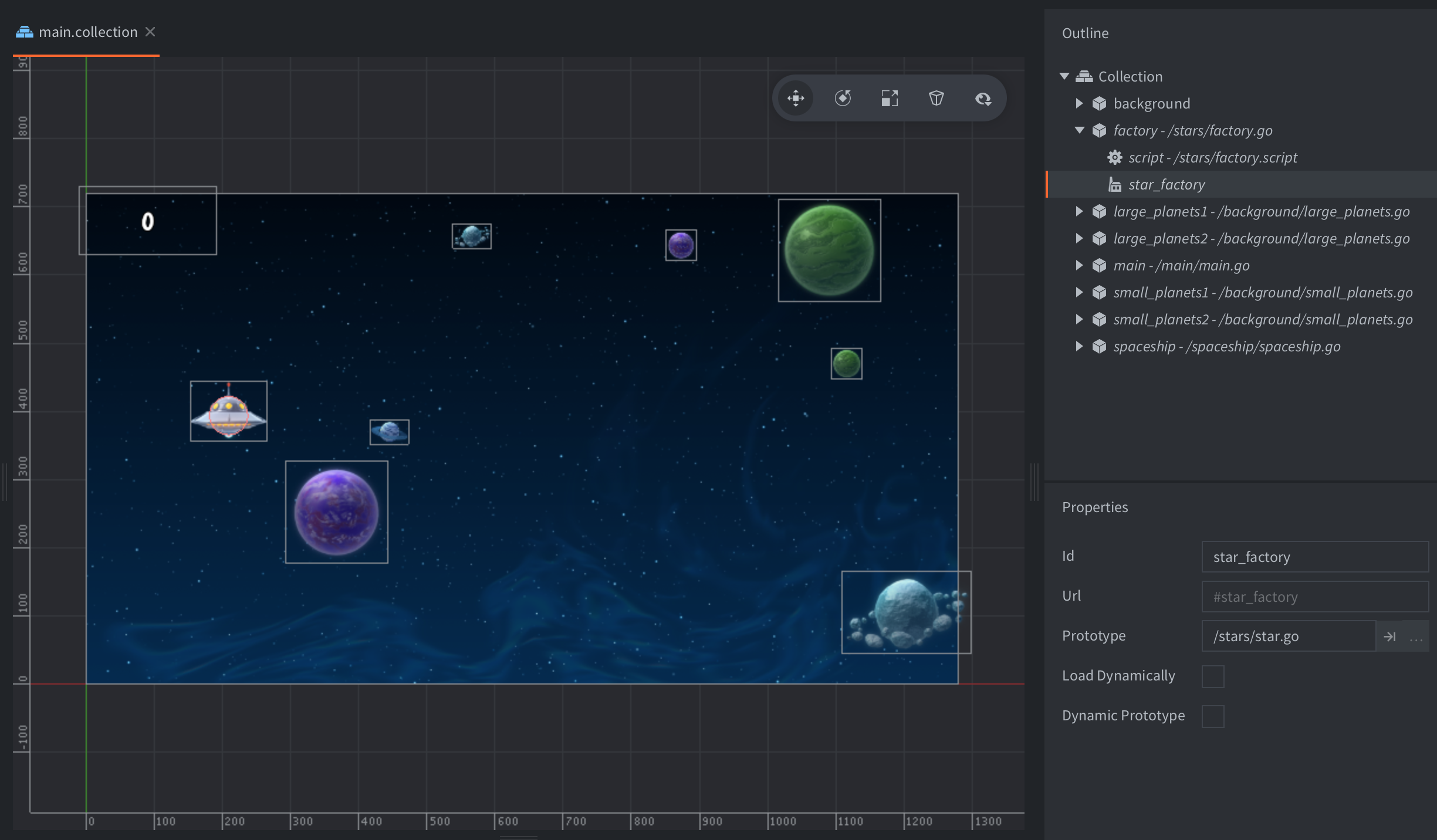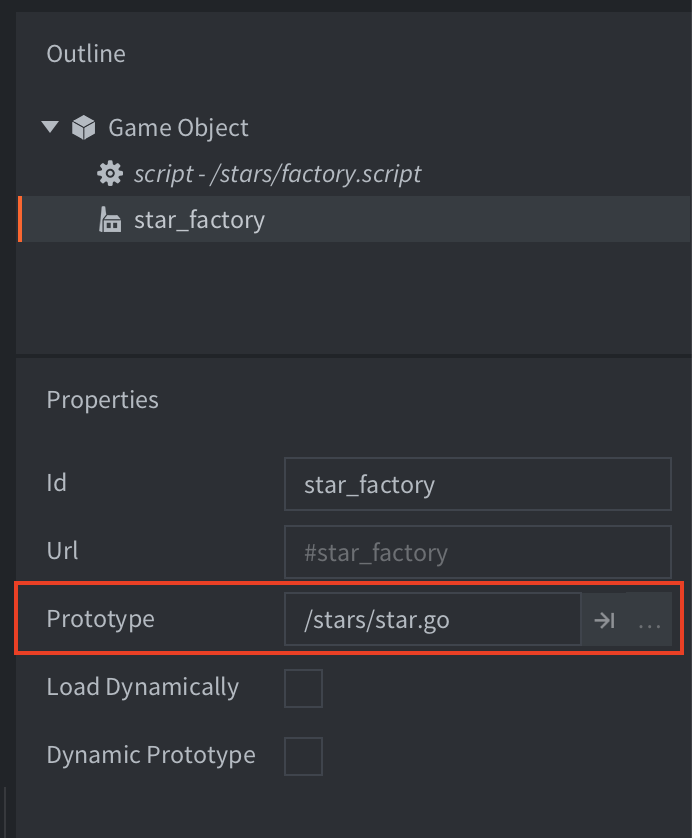``````-- factory.script
local p = go.get_position()
p.y = vmath.lerp(math.random(), min_y, max_y)
local component = "#star_factory"
factory.create(component, p)
``````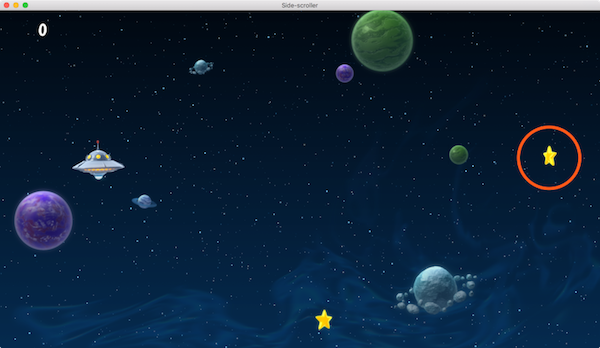`factory.create()` 有5个参数:

`url`

`[position]`
(可选) 新创建游戏对象的世界坐标位置. 以 `vector3` 表示. 如果不指定位置, 默认位置是工厂组件游戏对象的位置.
`[rotation]`
(可选) 新创建游戏对象的世界坐标旋转. 以 `quat` 表示.
`[properties]`
(可选) 新创建游戏对象的属性初始化 Lua 表. 详情请见 脚本属性教程.
`[scale]`
(可选) 新创建游戏对象的等比缩放. 以 `number` (大于 0) 表示. 或者以 `vector3` 表示每个坐标轴上的非等比缩放.

``````-- factory.script
local p = go.get_position()
p.y = vmath.lerp(math.random(), min_y, max_y)
local component = "#star_factory"
-- Spawn with no rotation but double scale.
-- Set the score of the star to 10.
factory.create(component, p, nil, { score = 10 }, 2.0) -- <1>
``````
1. 设置 star 游戏对象的 “score” 属性.
``````-- star.script
go.property("score", 1) -- <1>

local speed = -240

function update(self, dt)
local p = go.get_position()
p.x = p.x + speed * dt
if p.x < -32 then
go.delete()
end
go.set_position(p)
end

function on_message(self, message_id, message, sender)
if message_id == hash("collision_response") then
msg.post("main#gui", "add_score", {amount = self.score}) -- <2>
go.delete()
end
end
``````
1. “score” 脚本属性初始化时要有默认值.
2. “score” 脚本属性保存在 “self” 中.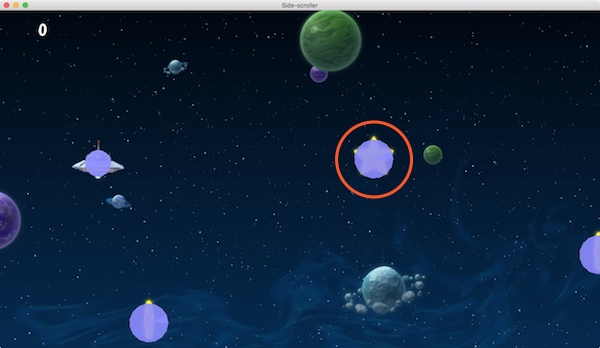Defold 目前不支持碰撞形状的非等比缩放. 如果赋了非等比值, 比如 `vmath.vector3(1.0, 2.0, 1.0)` 则 sprite 会正确缩放但是碰撞形状不会正确缩放.

## 定位新对象

Defold 的地址定位机制可以在运行时定位任何对象和组件. 关于定位详情请见 地址定位教程. 对于工厂新建对象及其组件同样如此. 新建的对象id经常被使用, 比如发送消息时:

``````local function create_hunter(target_id)
local id = factory.create("#hunterfactory")
msg.post(id, "hunt", { target = target_id })
return id
end
``````

``````local function create_guard(unarmed)
local id = factory.create("#guardfactory")
if unarmed then
local weapon_sprite_url = msg.url(nil, id, "weapon")
msg.post(weapon_sprite_url, "disable")

local body_sprite_url = msg.url(nil, id, "body")
sprite.play_flipbook(body_sprite_url, hash("red_guard"))
end
end
``````

## 新对象引用和对象父级

``````-- spawner.script
self.spawned_coins = {}

...

-- 把新建对象存入 "spawned_coins" 表中.
local id = factory.create("#coinfactory", coin_position)
table.insert(self.spawned_coins, id)
``````

``````-- coin.script
-- 删除所有 coins.
for _, coin_id in ipairs(self.spawned_coins) do
go.delete(coin_id)
end

-- 或者直接
go.delete(self.spawned_coins)
``````

``````-- spawner.script
-- 创建 drone 然后设置其引用者为此脚本
self.spawned_drone = factory.create("#dronefactory", drone_position, nil, { parent = msg.url() })

...

function on_message(self, message_id, message, sender)
self.spawed_drone = nil
end
end
``````

drone 的脚本:

``````-- drone.script
go.property("parent", msg.url())

...

function final(self)
-- 析构时.
end
``````

## 工厂资源的动态加载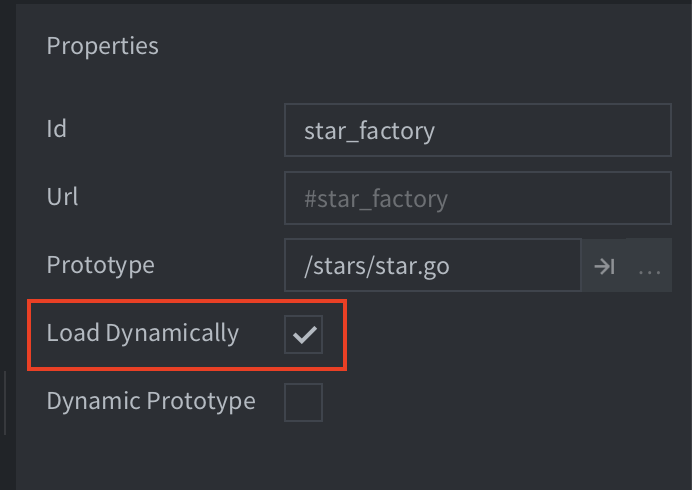``````function init(self)
-- 工厂父级集合加载时
-- 工厂资源不会被加载. 调用 create 函数
-- 会把资源进行同步加载.
self.go_id = factory.create("#factory")
end

function final(self)
-- 删掉游戏对象, 资源引用计数减少
-- 本例中工厂资源也会被卸载
-- 因为工厂组件不包含对资源的引用.
go.delete(self.go_id)

end
``````

``````function load_complete(self, url, result)
-- 资源加载完成, 可以新建对象
self.go_id = factory.create(url)
end

function init(self)
-- 工厂父级集合加载时
end

function final(self)
-- 删掉游戏对象, 资源引用计数减少
-- 本例中工厂资源不会被卸载
-- 因为工厂组件包含对资源的引用.
go.delete(self.go_id)

-- 这样资源才会被卸载.
end
``````

## 动态 Prototype

factory 组件里有 Dynamic Prototype 选项, 点选之后可以在运行时更改 factory 的原型.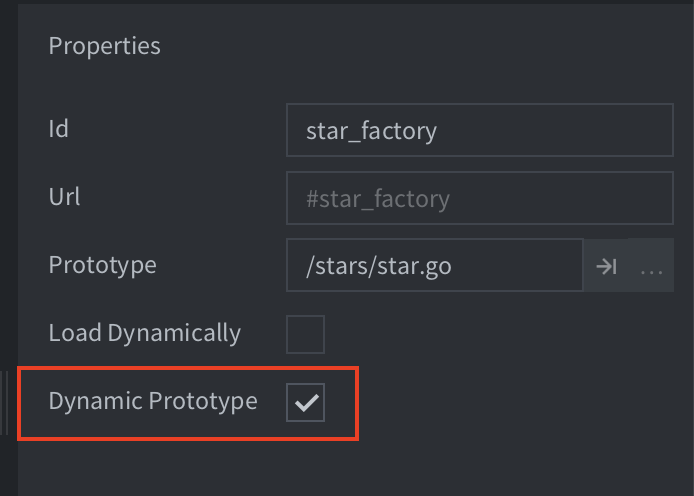factory 组件 Dynamic Prototype 被点选之后可以使用 `factory.set_prototype()` 函数更改其原型. 例如:

``````factory.unload("#factory") -- 卸载之前的资源
factory.set_prototype("#factory", "/main/levels/enemyA.goc")
local enemy_id = factory.create("#factory")
``````

Dynamic Prototype 被点选后, 集合组件数目将失去限制, factory 所在的集合将使用 game.project 文件指定的默认组件限制数目.

## 实例限制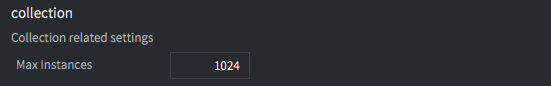## 游戏对象池

Did you spot an error or do you have a suggestion? Please let us know on GitHub!